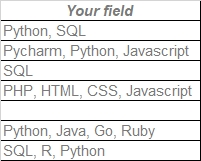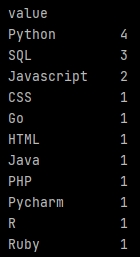### Analyze data from a multi-valued fieldSuppose you have a field containing values coming from a multi-valued drop-down list. But now you want to count how many times each value exists.

If values were uniques in each cell, a simple `GROUP BY` in SQL would do the job.

But here we first have to split each cell to recover unique values, then we will be able to count them.

#Pandas

#### Split the data

Start splitting the data with `str.split` specifying the separator and expanding, to create new columns to store each unique values.

```import pandas as pd

sheet_name='Sheet1', engine='openpyxl')

serie = df['Your field'].str.split(', ', expand=True)

print(serie)```

Great! Now we get virtual columns containing only one value, with as much columns as necessary.

#### Melt the virtual data

Now let's go to melt our virtual columns in just one.

```import pandas as pd

sheet_name='Sheet1', engine='openpyxl')

serieMelt = pd.melt(df['Your field'].str.split(', ',expand=True))['value']

print(serieMelt)```

`str.split` gives virtual column labels named `value`, that we can use.

#### Count unique values

Now just put our melted data in a new data frame, before to apply a sorted `groupby` by `size()` (a `COUNT` in SQL).The full code will be:

```import pandas as pd

temp_df = pd.DataFrame(pd.melt(df['Your field'].str.split(', ', expand=True))['value'])

df_Count = pd.DataFrame(temp_df.groupby(['value']).size(), columns=['Total']).reset_index()

print(df_Count)```

We add a `reset_index()` to recover column names properly, to use them later.

#### Percentage

Ok, but suppose you want also the recurrence percentages of your unique values. The usual method will take the total from the melted data, giving strange results. Indeed the melted data cumulated each values from the multi-valued field in new rows, so a lot!

To get real percentages you have to use `shape` to count the real number of rows from your original data-frame, and use it in the percentage calculation.

We start creating a new field in the results data-frame, then we calculate percentages, rounding them.

```df_Count['Percent'] = (df_Count['Total'] / df.shape) * 100
df_Count['Percent'] =df_Count['Percent'].round(decimals=2)```

Of course, you can not add the percentage results, it will exceed 100% and it is normal.

#### Empty values management

Good. But about our empty values? Just add `dropna=False` to see them.

```import pandas as pd

temp_df = pd.DataFrame(pd.melt(df['Your field'].str.split(', ', expand=True))['value'])
df_Count = pd.DataFrame(temp_df.groupby(['value'], dropna=False).size(), columns=['Total']).reset_index()

df_Count['Percent'] = (df_Count['Total'] / df.shape) * 100
df_Count['Percent'] =df_Count['Percent'].round(decimals=2)

print(df_Count)```

Any problem?

Arrgg! The `groupby` counted empty values from the melted data, and the melted data has added new columns as many times as necessary (based on the record with the highest number of unique values). Each them containing empty values ... It skews the count and percentage.

We have to cheat to fix that.

`import pandas as pd df = pd.read_excel('C:/Users/Georges/Downloads/My Excel file.xlsx', sheet_name='Sheet1', engine='openpyxl') temp_df = pd.DataFrame(pd.melt(df['Your field'].str.split(', ', expand=True))['value'])df_Count = pd.DataFrame(temp_df.groupby(['value'], dropna=False).size(), columns=['Total']).reset_index()df_Count = df_Count.fillna('AZERTY') df_Count['Percent'] = (df_Count['Total'] / df.shape) * 100df_Count['Percent'] =df_Count['Percent'].round(decimals=2) valuesEmpty = df['Industries:Industries'].isna().sum()valuesEmptyPercent = round((valuesEmpty / df.shape) * 100, 2) df_Count.loc[(df_Count['value'] == 'AZERTY')] = [['Unknow', valuesEmpty, valuesEmptyPercent]]df_Count = df_Count.sort_values(['Total'], ascending=False) print(df_Count)`

The 7th line adds a specific value in the results data-frame to target the wrong empty values calculation (`AZERTY`).

The 12 and 13th count the empty values and its percentage aside, and store results in specific variables.

Then the 15th line replaces the empty values calculation with our new value and variables, using `loc` to identify the line to fix.

Finally we can sort our data-frame with `sort_values(['Total']`.